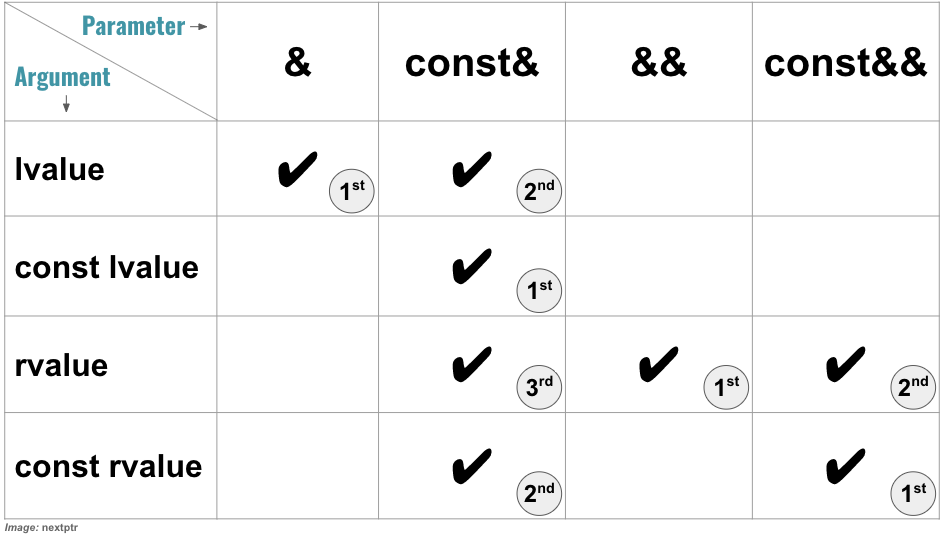# Beware of using std::move on a const lvalue

Unintentionally using std::move on a const lvalue will result in a copy where a move is intended.

With all due respect to its virtues, IMO, the move semantics is easily one of the most complicated subjects to grasp in C++.

In this article, we are presenting one of those situations where std::move is misapplied. Suppose, Blob (shown below) is a class that holds some data, and a copy-constructor, a move-constructor, a copy-assignment-operator, and a move-assignment-operator are customarily implemented for it:

``````class Blob {
public:
Blob() = default;

Blob(const Blob& rhs) {
// do copy
}
Blob(Blob&& rhs) {
//do move
}

//copy and move assignment operators..

//more methods ...

private:
// some data
};
``````

A function foo (shown below) takes a const lvalue reference of Blob as a parameter for read-only processing of data:

``````void foo(const Blob& blob) {
// process blob
}
``````

Someplace else in code, the foo is called, and the Blob argument is moved to an std::vector for storage:

``````// std::vector<Blob> v;
// Blob b;

foo(b);
// move to vector
v.push_back(std::move(b)); //moved
``````

At some point, it is determined that the code that moves the Blob to the std::vector should be transferred to foo as well. So, the foo is changed to take an std::vector< Blob>& parameter, but the parameter blob is inadvertently left `const`:

``````// 'blob' is still const
void foo(const Blob& blob, std::vector<Blob>& v) {
// process blob
v.push_back(std::move(blob)); //copied
}

// now foo is called as
// std::vector<Blob> v;
// Blob b;
foo(b,v);
``````

Everything works quietly as before, except that the Blob is no longer moved but is copied to the std::vector. The reason behind the copy as opposed to the move is that the parameter blob is `const`. To ensure that the blob is moved, the function foo should be fixed to take `Blob&` instead of `const Blob&`. But the question is, how can the const-ness of that lvalue interfere with the move mechanism?

The std::move guarantees to cast its parameter to an rvalue, but it does not alter the const-ness of the parameter. So, the outcome of applying std::move on a `const Blob&` is `const Blob&&`. In words, a const lvalue is cast into a const rvalue. However, an `std::vector<T>` does not have any push_back method that takes a `const T&&` parameter. These are all the two overloads of push_back method an `std::vector<T>` has:

``````//1. copy-constructor of T is called
void push_back(const T& t); //copies t to new element

//2. move-constructor of T is called
void push_back(T&& t); //moves t to new element
``````

Therefore the compiler, to respect the const-ness of the argument, chooses the std::vector< Blob>::push_back(const Blob&) method, which in turn invokes the copy-constructor of Blob.

To dig into it a little bit more, consider the following table that summarizes which type of parameter binding - `&`, `const&`, `&&`, `const&&` - can be bound to which value category of argument - lvalue, const lvalue, rvalue, const rvalue:Note that, more than one parameter binding type can be bound to a category of argument in well-defined preference order, shown as 1st or 2nd or 3rd in the table. As an example, the parameter binding `&` is preferred over the parameter binding `const&` for an lvalue argument. Following code shows some examples based on the above table:

``````void f1(std::string& s);
void f2(const std::string& s);
void f3(std::string&& s);
void f4(const std::string&& s);

std::string s("Hi"); //lvalue
const std::string cs("Hi"); //const lvalue

f1(s); //OK
f1(cs); //ERROR
f1(std::move(s)); //ERROR
f1(std::move(cs)); //ERROR

f2(s); // OK
f2(cs); //OK
f2(std::move(s)); //OK
f2(std::move(cs)); //OK

f3(s); //ERROR
f3(cs); //ERROR
f3(std::move(s)); //OK
f3(std::move(cs)); //ERROR

f4(s); //ERROR
f4(cs); //ERROR
f4(std::move(s)); //OK
f4(std::move(cs)); //OK
``````

The most important takeaway from the above table is that the `const&` can be bound to lvalues and rvalues, which makes the `const&&` parameter pointless. For rvalues we have `&&` parameter binding and for const rvalues we can use `const&` binding. That shows why we never see any functions taking `const&&`, and why the copy-constructor with `const&` parameter is invoked for the const rvalue result of the std::move above.